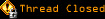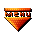# Preserved Topic: Bit vector -> array algorithm (Page 1 of 1)jiblet
Paranoid (IV) Inmate

From: Minneapolis, MN, USA
Insane since: May 2000posted 05-14-2001 20:24

So I wrote this function:

code:
```function vectorToArray(\$vector) {
\$bit = 2;
\$j = 1;
while (\$bit < \$vector) {
\$bit *= 2;
\$j++;
}
while (\$vector > 0) {
\$j--;
\$bit /= 2;
if (\$vector >= \$bit) {
\$bits[\$j] = 1;
\$vector -= \$bit;
} else {
\$bits[\$j] = 0;
}
}
return \$bits[\$j];
}```

Is there a better way to get a bit vector into an array (either a boolean array like I have, or else an int array with just the true bits)? I searched the PHP manual for a function that does something of this sort, but didn't find anything.

-jiblet

[This message has been edited by jiblet (edited 05-14-2001).]

WarMage

From: Rochester, New York, USA
Insane since: May 2000posted 05-14-2001 22:16

Mind giving an example of the usage. And a result. I can see how the code works, but I can't picture what you are exactly trying to do. The example would solve all confusion.linear
Paranoid (IV) Inmate

From: other places
Insane since: Mar 2001posted 05-14-2001 22:26

The beauteous thing about your vectors is using the bitwise operations. You set bits with OR, you read them with AND.

Customer picks 3 items out of possible eight from the form on your site. They have values that are positional:

0000 0001 1
0000 0010 2
0000 0100 4
0000 1000 8
0001 0000 16
0010 0000 32
0100 0000 64
1000 0000 128

//Customer orders item #32:
\$choices

jiblet
Paranoid (IV) Inmate

From: Minneapolis, MN, USA
Insane since: May 2000posted 05-14-2001 23:29

Ah I see. Very cool. I think my function still may have some use however. I've modified it so it returns an array of ints.

code:
```function vectorToArray(\$vector) {
\$bit = 2;
\$j = 1;
while (\$bit < \$vector) {
\$bit *= 2;
\$j++;
}
while (\$vector > 0) {
\$j--;
\$bit /= 2;
if (\$vector >= \$bit) {
array_push(\$bits, \$j);
\$vector -= \$bit;
}
}
return \$bits;
}```

This comes in handy for the code that populates the junction table of a many to many relationship:

[CODE]
\$result = mysql_query("DELETE FROM Event_Sponsor WHERE event_id=\$id");
foreach (\$bits as \$s) {
mysql_query("INSERT INTO Event_Sponsor VALUES (\$id, \$s)");
}
\$result = mysql_query("DELETE FROM Event_Ticketseller WHERE event_id=\$id");
\$bits = vectorToArray(\$ticketSellerIDs);
foreach (\$bits as \$s) {
mysql_query("INSERT INTO Event_Ticketseller VALUES (\$id, \$s)");
}
[\CODE]

I totally see your point linear, I should probably use your method to optimize my code. I kinda like this way because it simplifies the main portion a lot. The database uses 1 thru 8 rather than 2^0, 2^1... 2^8, so this way might be less error prone for the initial version, I can always update later.

PS. anyone know why the 2nd code tags didn't work?

[This message has been edited by jiblet (edited 05-14-2001).]

[This message has been edited by jiblet (edited 05-14-2001).]

linear
Paranoid (IV) Inmate

From: other places
Insane since: Mar 2001posted 05-15-2001 01:37

you closed with a backslash, not slash.

WarMage

From: Rochester, New York, USA
Insane since: May 2000posted 05-15-2001 02:30

In essence you are preforming Decimal to Binary Conversions.

code:
```public static String convert(int n)
{
String num = "";
while (n>0)
{
num = ""+n%2+""+num;
n = n >> 1;
}
return num;
}```

But instead of storing them in a String you would want the numbers stored in an array.

code:
```/*
* precondition: n < 255
* postcondition: return an array
* of type int with the binary
* value of n stored in it.
*/
public static int[] convert(int n)
{
int[] num = new int;
int i = 7;
while (n>0)
{
num[i] = n%2
n = n >> 1;
i--;
}
return num;
}```

Now you should have the values stored in the array, it starts from the back an moves forward, the array should have values of 0 stored in all empty locations, if not you will have to initialize the array with a simple for loop. If the precondition fails you will have an array out of bounds exception, which can be checked for, but it would be better to make the code follow so that exceptions are not able to result.

I have never used linear's method. But his code tends to be very compact and to the point, so I would trust his instincts.

The code I did was in Java, but it could be easily molded for php, if you simply turn the bitshift into a /= 2. I am not sure if PHP supports bit shifts, but they a faster if used correctly, but the speed gain is always minimal. I like using a string for this type of work because it uses much less memory, but it is harded to access correct portions.

But I will think that it would work as a for loop to fill the order for each bit.jiblet
Paranoid (IV) Inmate

From: Minneapolis, MN, USA
Insane since: May 2000posted 05-15-2001 22:59

Thanks warmage, PHP makes things much simpler because you never declare anything, just start filling the array. Yes, PHP does have bitshift, and I should have thought of using that. Your code appears to do more efficiently exactly what my original code did. I realized that a boolean array does not provide added value.

The function I am using now returns an array filled only with the numbers of the bits that are true. This makes for maximum efficiency because i can use those values directly in my SQL queries and the loop only runs as many times as there is values to be added to the junction table. I still may be able to use your tip to maximize efficieny though, thanks.

-jiblet